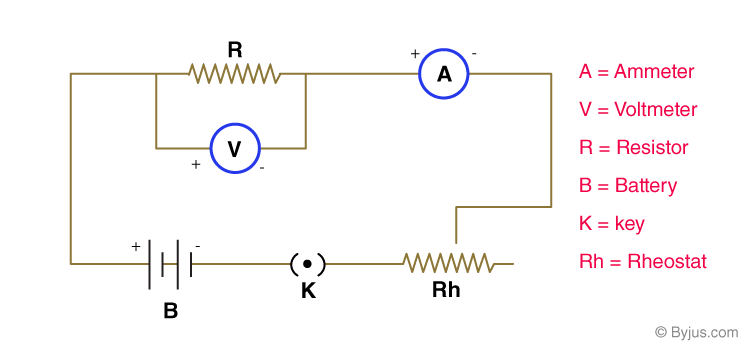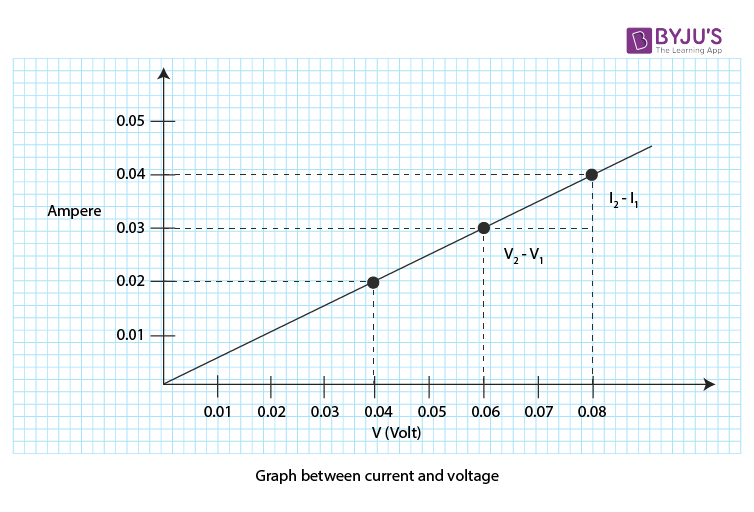# Studying the dependence of potential difference (V) across a resistor on the current (I) passing through it and determine its resistance. Also plotting a graph between V and I

Potential difference is defined as the work done to move a unit charge from one point to the other. The SI unit of potential difference is volt. Electromotive force is defined as the force that distributes the equilibrium of free-flowing electrons. Below is an experiment to study the dependence of the potential difference across a resistor with current-carrying I.

## Aim

To study the dependence of potential difference (V) across a resistor on the current (I) passing through it and determine its resistance. Also, plot a graph between V and I.

## Theory

### What is Ohm’s law?

In an electric circuit, the potential difference V across the metallic wire is directly proportional to the current flowing through the circuit with a constant temperature. This is known as Ohm’s law.

V∝I. ∴ V=IR.

### What are the factors affecting resistance?

Following are the factors affecting resistance:

• The nature of the resistor.
• With an increase in length, the resistance also increases. So length also affects the resistance.
• With an increase in the cross-sectional area, the resistance decreases. So cross-sectional area of the wire affects the resistance.

## Materials Required

Following is the list of materials required for this experiment:

1. A battery
2. An insulated copper wire
3. A key
4. An ammeter
5. A voltmeter
6. A rheostat
7. A resistor
8. A piece of sandpaper

### Circuit Diagram## Procedure

1. Arrange the devices as shown in the circuit diagram.
2. Connect the devices with the connecting wires keeping the key open.
3. The positive terminal of the battery should be connected to the positive terminal of the ammeter.
4. Before connecting the voltmeter in the circuit, check for +ve and -ve terminals.
5. Check for ammeter and voltmeter reading once the circuit is connected and also adjust the slider of rheostat after inserting the key.
6. For current I and voltmeter V, record three different readings using a slider.
7. Record the observations in the observation table.
8. Using the formula R=V/I, calculate the resistance.
9. To plot the graph between V and I, take V on the x-axis and I on the y-axis.
10. For pure metals, resistance increases with an increase in temperature.

## Observation Table

### i) Least count of ammeter and voltmeter

 Sl.no Ammeter (A) Voltmeter (V) 1 Range 0-0.5 A 0-0.1 V 2 Least count 0.01 A 0.01 V 3 Zero error (e) 0 0 4 Zero correction 0 0

### ii) For the reading of ammeter and voltmeter

 Sl.no Current in Ampere (I) (ammeter reading) Potential difference in volts (V) (voltmeter reading) Resistance in ohms R = V/I (Ω) Observed Corrected Observed Corrected 1 0 0.02 0 0.04 R1= 2Ω 2 0 0.03 0 0.06 R2= 2Ω 3 0 0.04 0 0.08 R3= 2Ω

## Graph## Conclusions

1. For all the three readings, the R-value is the same and constant.
2. The ratio of potential difference V and current I is the resistance of a resistor.
3. With the help of the graph between V and I, Ohm’s law is verified as the plot is a straight line.

## Precautions

1. Thick copper wires are used as connecting wires and using sandpaper, their insulation is removed.
2. To avoid external resistance, the connections should be tight.
3. The connections should be as per the circuit diagram and should be approved by the teacher before conducting the experiment.
4. The current should enter from the positive terminal and exit from the negative terminal of the ammeter and should be connected in series with the resistor.
5. Resistor and voltmeter should be connected in parallel.
6. The least count of ammeter and voltmeter should be recorded properly.
7. When there is no current flow, the pointers of ammeter and voltmeter should be at zero.
8. To avoid unnecessary heating in the circuit, the current should be passed for a short time.

## Viva Questions

Q1. Define electric current.

Ans: Electric current is defined as the rate of flow of electric charge in a conductor.

 $$I=\frac{Q}{t}$$

Where,

• I is the current in amperes
• Q is the electric charge in coulombs
• t is the time in seconds

Q2. What is the value of charge in 1 electron?

Ans: The value of charge in 1 electron is 1.6×10-19C.

Q3. What is coulomb?

Ans: Coulomb is an SI unit of electric charge and is defined as the amount of charge present in 6.25×1018 electrons.

Q4. What is 1 volt?

Ans: 1 volt is defined as the work done in 1 Joule to move a charge of 1 Coulomb from one point to the other than the potential difference is 1 volt.

Q5. What is 1 ohm?

Ans: 1 ohm is defined as the resistance offered by an object when one ampere current flows through an object with a potential difference of one volt.